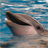# KSDK v2 SAI driver problem

キャンセル

もしかして:

## KSDK v2 SAI driver problem

1,540件の閲覧回数Contributor II

Dear Community,

I have following problems with SAI examples on my board (custom).

Configuration is:

KSDK build with online builder: SDK_2.0_MK26FN2M0xxx18

Demo runs under FreeRTOS (I copied examples code to created sound task).

Hardware: Kinetis K26, XTAL 8MHz run at 180MHz in PEE mode, codec - SGTL5000.

Clocks are configured as followed:

CLOCK_SetSimSafeDivs();

oscConfig.freq = BOARD_XTAL0_CLK_HZ;

oscConfig.workMode = kOSC_ModeOscLowPower;

oscConfig.oscerConfig.enableMode = kOSC_ErClkEnable;

CLOCK_InitOsc0(&oscConfig);

CLOCK_SetXtal0Freq(BOARD_XTAL0_CLK_HZ);

g_pllConfig.enableMode = 0U;

CLOCK_CalcPllDiv(g_xtal0Freq, BOARD_MCGOUTCLK_FREQ, &g_pllConfig.prdiv, &g_pllConfig.vdiv);

CLOCK_BootToPeeMode(kMCG_OscselOsc, kMCG_PllClkSelPll0, &g_pllConfig);

assert(kMCG_ModePEE == CLOCK_GetMode());

simConfig.pllFllSel = 1U;     /* PLLFLLSEL select PLL. */

simConfig.pllFllDiv = 0U;       /* PLLFLLSEL clock divider divisor */

simConfig.pllFllFrac = 0U;      /* PLLFLLSEL clock divider fraction */

simConfig.er32kSrc = 2U;        /* ERCLK32K selection, use RTC. */

simConfig.clkdiv1 = 0x02260000U; /* CKLDIV1 = 1,  CKLDIV2 = 3,  CKLDIV3 = 3,  CKLDIV4 = 7,  */

CLOCK_SetSimConfig(&simConfig);

SystemCoreClockUpdate();

I've checked all three examples (boards/twrk65f180m/driver_examples/sai ) and in all cases had some problems.

Ex 1 & 2.  interrupt & interrupt transfer

I can not play music.h clear at 180MHz. I heard some noises and after few playing loops like this

while (1)

{

PRINTF("Play ");

SAI_TransferSendNonBlocking(DEMO_SAI, &txHandle, &xfer);

/* Wait until finished */

isFinished = false;

/* Wait until finished */

while (isFinished != true)

PRINTF("Stop ");

}

it hangs. At 168MHz and lower everything runs ok for a long time.

Ex. 3 dma_transfer

Here I have the same problem with noises at coreClock >168MHz, moreover i can play sound only once

while (1)

{

PRINTF("Play ");

isFinished = false;

SAI_TransferSendEDMA(DEMO_SAI, &txHandle, &xfer);

/* Wait until finished */

while (isFinished != true)

PRINTF("Stop ");

}

it stopes on second isFinished loop.

What Am I doing wrong? Do I need any additional configuration?

ラベル(1)
• ### General

6 返答（返信）
713件の閲覧回数Contributor II

Solution to the Ex.3 appeared here:

How to reuse SAI_TransferSendEDMA in KSDK 2.0

Thank you.

713件の閲覧回数Contributor II

OK,

I found a solution.

The problem was with high speed mode.

If you want to use max CPU speed (180MHz in this case) you have to set high speed mode in the SMC module.

Like this (SMC->PMCTRL = ...)

Using KSDK 2.0 fsl_smc.h driver:

SMC_SetPowerModeProtection(SMC, kSMC_AllowPowerModeAll);

SMC_SetPowerModeHsrun(SMC);

while (SMC_GetPowerModeState(SMC) != kSMC_PowerStateHsrun)

{

}

And now sound works clear and stable.

Please if anyone could confirm my investigation, just write sth. here.

713件の閲覧回数Contributor II

One update.

It also run stable at 172MHz. Probably it's a PLL stability issue. I will change XTAL from 8 to 16 MHz and check again.

713件の閲覧回数NXP TechSupport

Hi, Jan,

As you described that the codec generates noise when the core/system frequency exceeds 168mHz, I suppose that the bit clock frequency of codec is incorrect.

I have checked both the code and the schematics of TWR-Audio-SGTL board, the SGTL5000 codec works in slave mode and synchronous mode, in other words, the Kinetis I2S interface genertates both the bit clock and frame clock, the receiver/transmitter of the codec use the same frame/bit clock. It also means that the core/system clock generates the bit/frame clock by a divider, the divider is only specified by the I2Sx_MDR.

for example, if you use 168MHz core/ssytem clock, the bit clock frequency is 32*2*16K=1.024MHz(sampling frequency is 16KHz), the diviedr should be 168MHz/1.024Mhz=164;

I suggest you set the core/system clock in 172MHz, in the case, if the set the divider as 168, the bit clcok will be 1.024Mhz approximatively.

I think you can write the I2Sx_MDR register dierctly with the code in  SAI_SetMasterClockDivider() in fsl_sai.c:

/* Fill the computed fract and divider to registers */

base->MDR = I2S_MDR_DIVIDE(167);   /// | I2S_MDR_FRACT(current_fract - 1);

If you want to research the divider, pls read the the function I pasted in fsk_sai.c,  but the code is not easy to understand:

You can also check the I2S_MDR register value in debugger.

BR

Xiangjun rong

static void SAI_SetMasterClockDivider(I2S_Type *base, uint32_t mclk_Hz, uint32_t mclkSrcClock_Hz)

{

uint32_t freq = mclkSrcClock_Hz;

uint16_t fract, divide;

uint32_t remaind = 0;

uint32_t current_remainder = 0xFFFFFFFFU;

uint16_t current_fract = 0;

uint16_t current_divide = 0;

uint32_t mul_freq = 0;

uint32_t max_fract = 256;

/*In order to prevent overflow */

freq /= 100;

mclk_Hz /= 100;

/* Compute the max fract number */

max_fract = mclk_Hz * 4096 / freq + 1;

if (max_fract > 256)

{

max_fract = 256;

}

/* Looking for the closet frequency */

for (fract = 1; fract < max_fract; fract++)

{

mul_freq = freq * fract;

remaind = mul_freq % mclk_Hz;

divide = mul_freq / mclk_Hz;

/* Find the exactly frequency */

if (remaind == 0)

{

current_fract = fract;

current_divide = mul_freq / mclk_Hz;

break;

}

/* Closer to next one, set the closest to next data */

if (remaind > mclk_Hz / 2)

{

remaind = mclk_Hz - remaind;

divide += 1;

}

/* Update the closest div and fract */

if (remaind < current_remainder)

{

current_fract = fract;

current_divide = divide;

current_remainder = remaind;

}

}

/* Fill the computed fract and divider to registers */

base->MDR = I2S_MDR_DIVIDE(current_divide - 1) | I2S_MDR_FRACT(current_fract - 1);  //Rong wrote, change the value here directly

/* Waiting for the divider updated */

{

}

}

#endif /* FSL_FEATURE_SAI_HAS_MCLKDIV_REGISTER */

713件の閲覧回数Contributor II

I've checked under debugger.

@120MHz FRACT = 32, DIVIDE = 625, so clock is exact 6,144MHz

@168MHz FRACT = 32, DIVIDE = 875, exact 6,144MHz

@180MHz FRACT = 64, DIVIDE = 1875, same 6,144MHz

So, the function you paste is OK.

As you suggest I set clock/system speed at 180MHz

and SAI clock settings

base->MDR = I2S_MDR_DIVIDE(29);

... and still noise.

713件の閲覧回数Contributor II

OK,

I will check it.